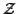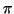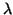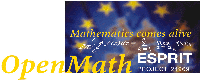Next: OpenMath and MathML Up: The OpenMath Project Final Previous: Technical Summary   Contents

Subsections

# The OpenMath Standard

A lot of the mathematics online has until recently been presented using the de-facto standard LaTeX (or TeX) despite the well known limitations it has in handling interactivity. Documents generated by transforming TeX sources to various flavors of HTML support only cross-linking mechanisms and produce, in most cases, images for formulae. More adventurous authors have included interactivity in the form of plug-ins or JAVA applets in their pages. In the past three years things have changed and new web technologies have established themselves. In particular, the World Wide Web Consortium has published the recommendation for MathML, the Mathematical Markup Language for describing mathematical notation and capturing both its structure and content . Similarly, the OpenMath Society has endorsed a newer version of the OpenMath Standard for representing the semantics of mathematical objects and facilitating the exchange between computer programs, the storage in knowledge bases, and the electronic publication of mathematics . In this document we describe the OpenMath Standard.

By enabling mathematics to be served, received, and processed electronically, both MathML and OpenMath aim at enhancing mathematical communication on the on the World Wide Web just as hypertext has done for text. The parallel evolution and definition of these predominant markup languages for mathematics  resulted in an ideal separation of complementary rôles: MathML presentation can be used for presenting mathematical content written in OpenMath. The major observation underlying this state of affairs is that mathematics and its presentation should not be viewed as one and the same thing. While the meaning of a mathematical object should be uniquely defined and understood, its visualisation and rendering depends on time and place, more precisely it depends on the context and on the style preferences of the author or reader.

OpenMath is a language for representing and communicating mathematics . Originally, it was conceived as a language for all computer algebra packages [26,23]. Presently it is equipped for conveying mathematical concepts arising from all areas of mathematics, for instance logic. Now OpenMath can be used to express formal mathematical objects, so that formal theorems and proofs, understandable to proof checkers, can be communicated, as well as the usual mathematical expressions handled by computer algebra packages. In order to represent mathematical content it is crucial that a mechanism is established to allow for the introduction of new concepts, since this activity is at the core of mathematics. The OpenMath representation of mathematics relies for this task on a small set of expression tree'' constructors (application, binding, attribution and error), on some basic objects (byte-arrays, strings, integers, IEEE floats, variables), and on the usage of symbols defined in Content Dictionaries. These are publicly available collections of mathematical definitions, a sort of xml dictionary of mathematics. The interested reader may find the latest versions of the standard documents and the collection of public Content Dictionaries at the OpenMath Society website.

There are several aspects of OpenMath. Those presented in this section are: the architecture of how OpenMath views integration of computational software, the OpenMath Standard, and the OpenMath Phrasebooks and tools. The OpenMath Standard is concerned with the objects, their encodings, and the Content Dictionaries. The reader is referred to the OpenMath Standard   for details.

# OpenMath Architecture

The architecture of OpenMath is made up of three layers of representation of a mathematical object: the private layer for the internal representation, the abstract layer for the representation as an OpenMath object, and the communication layer for translating the OpenMath object to a stream of bytes. An application will generally manipulate mathematical objects using its internal representation; it can convert them to OpenMath objects and communicate them by using the byte stream representation of OpenMath objects.

It is not within the scope of OpenMath to define how communication takes place, or the behaviour of services running in an integrated mathematical environment. OpenMath defines the structure and semantics of the objects being exchanged, not the actions taken when an object is received. For example, OpenMath can represent an integrand, and an algebra system might try to evaluate it in closed form while a renderer might attempt to print it on a screen. Therefore, OpenMath is one ingredient among many others that are needed for achieving integration of computational tools, see for example a description of how OpenMath is being used in recent applications .

OpenMath objects are representations of mathematical entities that can be communicated among various software applications in a meaningful way, that is in such a way that their semantics'' are well-defined. OpenMath provides basic objects like integers, symbols, floating-point numbers, character strings, byte-arrays, and variables, and compound objects: applications, bindings, errors, and attributions. Content Dictionaries (CDs) specify the meaning of symbols informally using natural language and, optionally, they might formally assign type information in the signature of the symbols. CDs are public and are used to represent the actual common knowledge among OpenMath-compliant applications. A central idea to the OpenMath philosophy is that CDs fix the meaning'' of objects independently of the application.

The integration of various software packages is achieved by means of OpenMath Phrasebooks; they convert OpenMath objects to and from the software package's internal representation and determine the package's actions. The translation is governed by the CDs and the specifics of the software packages.

# OpenMath Objects

We now focus on the abstract layer, where mathematical objects are represented by labelled trees, called OpenMath objects or OpenMath expressions. The formal definition of an abstract OpenMath object is given below.

Definition 4.2.1   OpenMath objects are built recursively as follows.

(i)
Basic OpenMath objects, e.g., integers, IEEE floating point numbers, Unicode character strings, byte-arrays, symbols (defined in CDs) and variables, are OpenMath objects.

(ii)
If A1, ..., An (n > 0) are OpenMath objects, then
application (A1,..., An)
is an OpenMath application object.

(iii)
If S1,..., Sn are OpenMath symbols, and A, A1, ..., An, (n > 0) are OpenMath objects, then
attribution (A, S1  A1,  ...  , Sn  An)
is an OpenMath attribution object and A is the object stripped of attributions. The operation of recursively applying stripping to the stripped object is called flattening of the attribution. When the stripped object after flattening is a variable, the attributed object is called attributed variable.

(iv)
If B and C are OpenMath objects, and v1, ..., vn (n0) are OpenMath variables or attributed variables, then
binding (B, v1,..., vn, C)
is an OpenMath binding object.

(v)
If S is an OpenMath symbol and A1, ..., An (n0) are OpenMath objects, then
error (S, A1,..., An)

is an OpenMath error object.

As mentioned earlier, OpenMath Content Dictionaries (CD) collect and provide definitions of mathematical notions for usage within OpenMath objects as described in Section 4.4. The official repository for CDs is . In this document we denote by foo:bar the symbol with name bar defined in the CD foo.

OpenMath abstract objects representing mathematical concepts are obtained for instance by using the application constructor as in the following examples.

Example 4.2.1   As a first example, consider the OpenMath object A corresponding to 1 + 2,

application(arith1:plus, 1, 2)

where arith1:plus is the symbol plus defined in the CD arith1 and 1 and 2 represent basic objects (arbitrary length integers).

Example 4.2.2   Applications can also be useful for representing algebraic structures. Consider the polynomial ringp[X]. It is representable as OpenMath abstract object like:
 application(polyr : PolynomialRingR,application(setname2 : Zm, p), x) (4.1)

In this object, the OpenMath symbols polyr:PolynomialRingR and setname2:Zm identify the polynomial ring structure obtained from the integers modulo p. More precisely, they are the symbols called PolynomialRingR and Zm defined in the CDs polyr and setname2, respectively.

Example 4.2.3   Now a slightly more complicated OpenMath object is given in Figure 4.1, namely the one for1 -dx.

It involves, amongst others, the CDs arith1 for the symbols e, +, -, *, /, ^ and nums1 forand imaginary i.# OpenMath Encodings

OpenMath encodings map OpenMath objects to byte streams that can easily be exchanged between processes or stored and retrieved from files.

Two major encodings supported and described by the OpenMath Standard are XML and binary. The first encoding uses only ISO 646:1983 characters  (ASCII characters) and is suitable for sending OpenMath objects via e-mail, news, cut-and-paste, etc. and for further processing by a variety of XML tools.

The second encoding is a custom-built binary encoding meant to be used when compactness is crucial, for instance in interprocess communications of very large objects over a network.

Example 4.3.1   For example, consider a polynomial with coefficients in the ring of integers modulo some prime p, f = X3 - X + 1. It can be represented in several ways as an abstract OpenMath object, either as univariate or (degenerate) multivariate, as a sum of terms or vector of coefficients etc. Supposing that we wish to represent f recursively, i.e. as a univariate polynomial whose coefficients are themselves univariate polynomials and so on, by using the symbol polyr:PolynomialR, It can be encoded in a human-readable format using XML and stored as:

<OMOBJ><OMA><OMS cd="polyr" name="PolynomialR"/>


<OMA><OMS cd="polyr" name="PolyRrep"/>
<OMV name="X"/>
<OMA><OMS cd="polyr" name="monomial"/>
<OMI> 3 </OMI><OMI> 1 </OMI>
</OMA>
<OMA><OMS cd="polyr" name="monomial"/>
<OMI> 1 </OMI><OMI> -1 </OMI>
</OMA>
<OMA><OMS cd="polyr" name="monomial"/>
<OMI> 0 </OMI><OMI> 1 </OMI>
</OMA>
</OMA>
</OMA>
</OMOBJ>

Reading from the encoding of the example, the outermost XML element <OMOBJ> encloses nested OpenMath application objects, appearing within the element <OMA>, which are built using OpenMath symbols (<OMS>), OpenMath variables (<OMV>), and integers (<OMI>). Notice that the application object highligted in bold above is essentially the XML encoding of the polynomial ring expressed abstractly in equation (4.1).

When embedding XML encoded OpenMath objects into a larger XML document one may wish, or need, to use additional features. For example use of extra XML attributes to specify XML Namespaces  or xml:lang attributes to specify the language used in strings . Also, the encoding used in the larger document may not be UTF-8. In particular, if OpenMath is used with applications that use the >XML Namespace Recommendation   then they should ensure that OpenMath elements are in the namespace http://www.openmath.org/OpenMath. This is most conveniently achieved by adding the namespace declaration xmlns="http://www.openmath.org/OpenMath" as an attribute to each OMOBJ element in the document.

# OpenMath Content Dictionaries

A Content Dictionary holds the meanings of (various) mathematical words' referred to as symbols. A set of official CDs, each covering a specific area has been produced and is available from the CD repository of the OpenMath society. Content Dictionaries may be grouped into CD groups, so that applications can easily refer to collections of Content Dictionaries. For instance, the MathML CD group covers essentially the same areas of mathematics as the content elements of the MathML recommendation .

While OpenMath objects are built using symbols defined in some Content Dictionary that is part of an ever growing collection of Content Dictionaries, MathML makes explicit a relatively small number of commonplace mathematical constructs chosen within the K-12 realm of applications and, in addition, it provides the content symbol (csymbol) to introduce a new symbol whose semantics is not part of the core content elements of MathML. In particular, such an external definition may reside in an OpenMath Content Dictionary as in the following example.

Example 4.4.1   Consider the OpenMath abstract object of Example 4.2.2. It can be expressed in MathML by introducing csymbol where an OpenMath symbol is needed in the encoding and is not supplied by the core MathML elements.

<apply>
<csymbol encoding="OpenMath" definitionURL="http://www.openmath.org/cd/polyr.ocd">
PolynomialR
</csymbol>
<apply>
<csymbol encoding="OpenMath" definitionURL="http://www.openmath.org/cd/setname2.ocd">
Zm
</csymbol>
<ci>p</ci>
</apply>
<ci>X</ci>
</apply>

MathML supports an extensive library of presentation symbols to accomodate even the fanciest notation used by mathematicians. In this respect, MathML (presentation) is the preferred choice for rendering mathematical content and as such it is to be hoped that it will be soon be supported by tools such as browsers and editors.

Users may submit their private CDs for public use. Before they can be adopted as official OpenMath CD they undergo a refereeing process by appointed members of the OpenMath Society. Details of this process along with advice and tips for CD authors are contained in the document On Writing Content Dictionaries.

CDs hold two types of information: that which applies to the whole CD (appears in the header of the CD), and that which is only relevant to a particular symbol definition (appears in a CD Definition). Information pertinent to the whole CD includes the name, a description, an expiration date, the status of the CD (official, experimental, private, obsolete), and an optional list of CDs on which it depends. Information restricted to a particular symbol includes a name and a description in natural language. Optional information includes examples of the use of this symbol, and formal properties satisfied by this symbol which can be expressed as OpenMath objects or as plain text.

A browsable version of the set of content dictionaries produced in the project can be found here, while the latest official CDs can be found on the OpenMath Society website.

## Signatures

Although OpenMath does not enforce formal specification of symbols by using signatures and axiomatic definitions, it recognizes their advantages and leaves room to adopt them if required. In particular, formal signatures in a specific type system can be used to assign mathematical meaning to OpenMath objects in such a way that validation of objects depends exclusively on the context determined by the CDs and on some type information carried by the objects themselves. Formal signatures and definitions are collected in additional files that can be associated to a Content Dictionary. Signatures of symbols are expressed in Signature files by OpenMath objects representing types in a certain type system.

### Light-weight Simple Type System

The Small Type System for OpenMath signatures has been designed according to two requirements.

• Tools which read new'' CDs automatically, e.g. a search engine which suddenly encountered as a symbol from a CD that it did not recognise. In the context of a Small'' type system, there is little more that can be offered to such systems than arity checking.
• Human beings reading the whole of a CD's fields, to determine how they ought to implement the CD, either as OpenMath-reading software, or, more interestingly, as OpenMath-writing software. These humans need to interpret the symbols in a way that cannot be totally formalised. However they ought to be'' imbued with that nebulous quality of common sense''.
To meet these goals, a system that encodes arity (and a little more) in a totally formal way, but leaves clues in names etc. that should help the human reader has been used, see A Small OpenMath Type System   for details.

### Calculus of Constructions

More formal signatures can also be associated to OpenMath symbols with the goal in mind of certifying the meaningfullness of OpenMath objects. For instance in , extensions of the Calculus of Constructions (CC) have been chosen as a starting point for assigning signatures to OpenMath symbols because they are expressive, well suited to modeling algebra [13,9,31], and have decidable type inference. Freely available software packages like Lego or COQ [30,25] have implemented the necessary functionality for performing type checks on OpenMath objects. For more details see A Type System For OpenMath.

## Defining Mathematical Properties

Axiomatic definitions in a specific formal system are expressed as OpenMath objects in DefMP (defining mathematical property) files. More details are to be found in .

Example 4.4.2   Consider a private CD, pock defining symbols needed in an application dealing with primality testing. Beside giving a natural language description, the author may introduce a new symbol pock:divides by a formal definition in terms of the DefMP representing the expressionn :.m :.(q :)  n = qm:


binding(lc:Lambda,
binding(quant1:exists,
application(relation1:eq,
n,
application(arith1:times, m, q))))
`

# OpenMath Phrasebooks

The programs that act as an interface between a software system and OpenMath are called phrasebooks. Their task is to translate the OpenMath object, as understood by means of Content Dictionaries, to the corresponding internal representation used by the specific software package.Phrasebooks providing an interface to and from OpenMath have been built into experimental versions of both AXIOM and GAP, cf. [21,22]. These are examples in which the software package itself provides the interface to and from OpenMath internally. A Mathematica phrasebook based on the OpenMath C library is available at INRIA.

Notice that the OpenMath phrasebooks are mainly concerned with the translation between OpenMath objects and internal system-specific representation. The interpreted behavior for most computer algebra packages receiving an OpenMath object is an evaluation. For instance, upon input of the object application(integer:rem, application(integer:gcd, 12, 3), 2) Mathematica would output 1.

In general, control of the interaction with a software package is not ruled by the existing OpenMath libraries. For this task, OpenMath allows freedom of choice between several paradigms [14,28].

Translation tools  have been produced which map between the common subset of mathematics covered by MathML-Content and the OpenMath CD group MathML. For displaying OpenMath objects with Presentation MathML, one may use xsl stylesheets driven by OpenMath CDs. Sample stylesheets are available from the OpenMath Society web pages.

## OpenMath Compliancy

OpenMath compliancy is desirable as a means to maximize the potential for interoperability amongst OpenMath applications. To be OpenMath compliant an application has to fullfill requirements dealing with the encoding it can accept or generate, with the declaration and handling of CDs it understands and with the error behaviour. The detailed description of compliancy is part of the standard  .Next: OpenMath and MathML Up: The OpenMath Project Final Previous: Technical Summary   Contents
The OpenMath Consortium